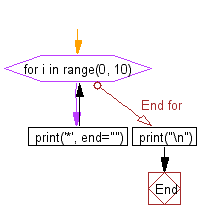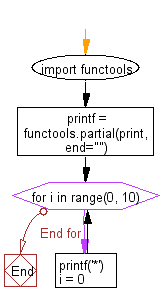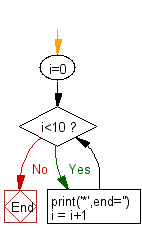﻿ Python: Print without newline or space - w3resource# Python: Print without newline or space

## Python Basic: Exercise-50 with Solution

Write a Python program to print without newline or space.

Sample Solution-1:

Python Code:

``````for i in range(0, 10):
print('*', end="")
print("\n")
```
```

Sample Output:

```**********
```

Flowchart:## Visualize Python code execution:

The following tool visualize what the computer is doing step-by-step as it executes the said program:

Sample Solution-2:

Python Code:

``````import functools
printf = functools.partial(print, end="")
for i in range(0, 10):
printf('*')
i = 0
```
```

Sample Output:

```**********
```

Flowchart:## Visualize Python code execution:

The following tool visualize what the computer is doing step-by-step as it executes the said program:

Sample Solution-3:

Python Code:

``````i=0;
while i<10 :
print('*',end='')
i = i+1
```
```

Sample Output:

```**********
```

Flowchart:## Visualize Python code execution:

The following tool visualize what the computer is doing step-by-step as it executes the said program:

Python Code Editor:

Have another way to solve this solution? Contribute your code (and comments) through Disqus.

What is the difficulty level of this exercise?

Test your Python skills with w3resource's quiz

﻿

## Python: Tips of the Day

Try-catch-else construct:

```try:
foo()
except Exception:
print("Exception occured")
else:
print("Exception didnt occur")
finally:
print("Always gets here")
```